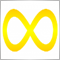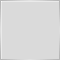# Operational confusion?23840

I cant see why the object "margin" is returning 0.0 and not 0.5 like it should?

```void OnTick()
{
double margin = (5 / 10);

Print("margin = ",margin);
}```Moderator
2312

Stephen Reynolds:

I cant see why the object "margin" is returning 0.0 and not 0.5 like it should?

```void OnTick()
{
double margin = (5 / 10.);

Print("margin = ",margin);
}```

Have a read here to find out why:

```   int    i=1/2;        // no types casting, the result is 0
Print("i = 1/2  ",i);

int k=1/2.0;         // the expression is cast to the double type,
Print("k = 1/2  ",k);  // then is to the target type of int, the result is 0

double d=1.0/2.0;    // no types casting, the result is 0.5
Print("d = 1/2.0; ",d);

double e=1/2.0;      // the expression is cast to the double type,
Print("e = 1/2.0; ",e);// that is the same as the target type, the result is 0.5

double x=1/2;        // the expression of the int type is cast to the double target typr,
Print("x = 1/2; ",x);  // the result is 0.0```22428